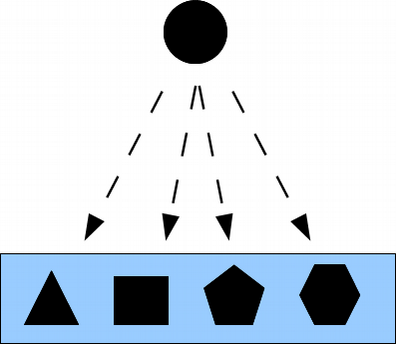# Partitions and Objective Indefiniteness in Quantum MechanicsClassical physics and quantum physics suggest two meta-physical types of reality: the classical notion of a objectively definite reality with properties “all the way down,” and the quantum notion of an objectively indefinite type of reality. The problem of interpreting quantum mechanics (QM) is essentially the problem of making sense out of an objectively indefinite reality. These two types of reality can be respectively associated with the two mathematical concepts of subsets and quotient sets (or partitions) which are category-theoretically dual to one another and which are developed in two dual mathematical logics, the usual Boolean logic of subsets and the more recent logic of partitions. Our sense-making strategy is “follow the math” by showing how the mathematics of set partitions can be transported in a natural way to complex vector spaces where it yields the mathematical machinery of QM. And then we show how the machinery of QM can be transported the other way down to set-like vector spaces over ℤ₂ yielding a rather fulsome “toy” or pedagogical model of “quantum mechanics over sets.” In this way, we try to make sense out of objective indefiniteness and thus to interpret quantum mechanics.